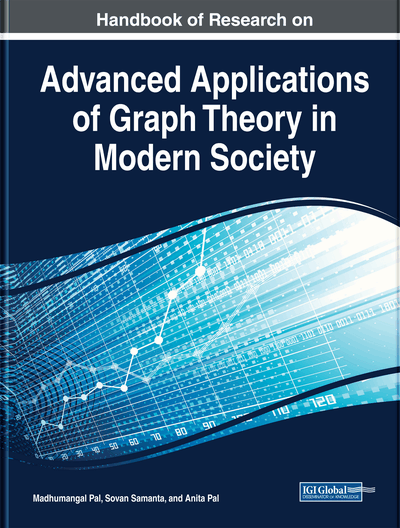# An Introduction to Intersection Graphs

DOI: 10.4018/978-1-5225-9380-5.ch002
Available
\$37.50
No Current Special Offers

## Abstract

In this chapter, a very important class of graphs called intersection graph is introduced. Based on the geometrical representation, many different types of intersection graphs can be defined with interesting properties. Some of them—interval graphs, circular-arc graphs, permutation graphs, trapezoid graphs, chordal graphs, line graphs, disk graphs, string graphs—are presented here. A brief introduction of each of these intersection graphs along with some basic properties and algorithmic status are investigated.
Chapter Preview
Top

## Introduction

The graphs are very useful tool to model a huge number of real life problems starting from science, technology, medical science, social science and many other areas. The geometrical and topological stuctures of any communication system such as Internet, Facebook, Whatsapp, ResearchGate, Twiter, etc. are based on graph. So graph theory is an old as well as young topic of research as till today graphs are used to solve several problems. Depending on the geometrical structures and properties different type of graphs are defined, viz. path, cycle, tree, complete graph, planar graph, perfect graph, chordal graph, tolarence graph, intersection graph, etc.

Table 1.
Table of abbreviations
 Abbreviation Description IntG Intersection graph InvG Interval graph CirG Circular-arc graph PerG Permutation graph TraG Trapezoidal graph TolG Tolerance graph PCA Proper circular-arc UCA Unit circular arc GIG Grid intersection graphs

In this chapter, diferent types of intersection graphs (IntGs) are defined and investigated their properties.

.

The resultant graph is called intersection graph.

## Complete Chapter List

Search this Book:
Reset